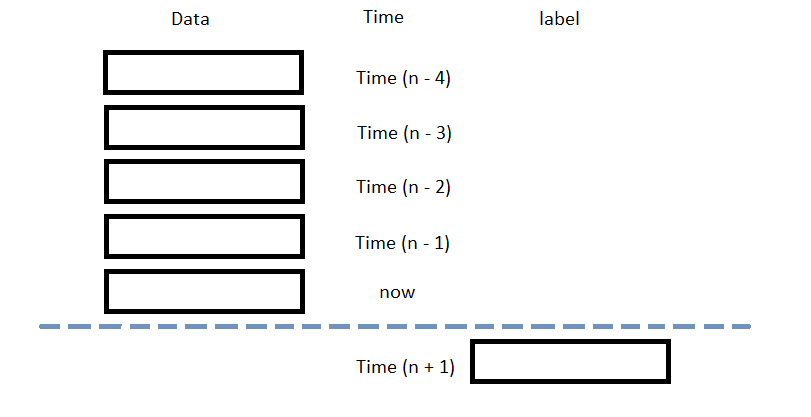# Popular Libraries

## PyTorch

### Introduction

This page explains how to build, train, deploy and store PyTorch models.

### Import Libraries

Import the torch and joblib libraries.

from AlgorithmImports import *
import torch
from torch import nn
import joblib

You need the joblib library to store models.

### Create Subscriptions

In the Initialize method, subscribe to some data so you can train the torch model and make predictions.

self.symbol = self.AddEquity("SPY", Resolution.Daily).Symbol

### Build Models

In this example, build a neural-network regression model that uses the following features and labels:

Data CategoryDescription
FeaturesThe last 5 closing prices.
LabelsThe following day's closing price

The following image shows the time difference between the features and labels:Follow these steps to create a method to build the model:

1. Define a subclass of nn.Module to be the model.
2. In this example, use the ReLU activation function for each layer.

class NeuralNetwork(nn.Module):
# Model Structure
def __init__(self):
super(NeuralNetwork, self).__init__()
self.flatten = nn.Flatten()
self.linear_relu_stack = nn.Sequential(
nn.Linear(5, 5),   # input size, output size of the layer
nn.ReLU(),         # Relu non-linear transformation
nn.Linear(5, 5),
nn.ReLU(),
nn.Linear(5, 1),   # Output size = 1 for regression
)

# Feed-forward training/prediction
def forward(self, x):
x = torch.from_numpy(x).float()   # Convert to tensor in type float
result = self.linear_relu_stack(x)
return result
3. Create an instance of the model and set its configuration to train on the GPU if it's available.
4. device = 'cuda' if torch.cuda.is_available() else 'cpu'
self.model = NeuralNetwork().to(device)

### Train Models

You can train the model at the beginning of your algorithm and you can periodically re-train it as the algorithm executes.

#### Warm Up Training Data

You need historical data to initially train the model at the start of your algorithm. To get the initial training data, in the Initialize method, make a history request.

training_length = 252*2
self.training_data = RollingWindow[float](training_length)
self.training_data.Add(trade_bar.Close)

#### Define a Training Method

To train the model, define a method that fits the model with the training data.

def get_features_and_labels(self, n_steps=5):
close_prices = list(self.training_data)[::-1]

features = []
labels = []
for i in range(len(close_prices)-n_steps):
features.append(close_prices[i:i+n_steps])
labels.append(close_prices[i+n_steps])
features = np.array(features)
labels = np.array(labels)

return features, labels

def my_training_method(self):
features, labels = self.get_features_and_labels()

# Set the loss and optimization functions
# In this example, use the mean squared error as the loss function and stochastic gradient descent as the optimizer
loss_fn = nn.MSELoss()
learning_rate = 0.001
optimizer = torch.optim.SGD(self.model.parameters(), lr=learning_rate)

# Create a for-loop to train for preset number of epoch
epochs = 5
for t in range(epochs):
# Create a for-loop to fit the model per batch
for batch, (feature, label) in enumerate(zip(features, labels)):
# Compute prediction and loss
pred = self.model(feature)
real = torch.from_numpy(np.array(label).flatten()).float()
loss = loss_fn(pred, real)

# Perform backpropagation
loss.backward()
optimizer.step()

#### Set Training Schedule

To train the model at the beginning of your algorithm, in the Initialize method, call the Train method.

self.Train(self.my_training_method)

To periodically re-train the model as your algorithm executes, in the Initialize method, call the Train method as a Scheduled Event.

# Train the model every Sunday at 8:00 AM
self.Train(self.DateRules.Every(DayOfWeek.Sunday), self.TimeRules.At(8, 0), self.my_training_method)

#### Update Training Data

To update the training data as the algorithm executes, in the OnData method, add the current TradeBar to the RollingWindow that holds the training data.

def OnData(self, slice: Slice) -> None:
if self.symbol in slice.Bars:
self.training_data.Add(slice.Bars[self.symbol].Close)

### Predict Labels

To predict the labels of new data, in the OnData method, get the most recent set of features and pass it to the model.

features, __ = self.get_features_and_labels()
prediction = self.model(features[-1].reshape(1, -1))
prediction = float(prediction.detach().numpy()[-1])

You can use the label prediction to place orders.

if prediction > slice[self.symbol].Price:
self.SetHoldings(self.symbol, 1)
elif prediction < slice[self.symbol].Price:
self.SetHoldings(self.symbol, -1)

### Save Models

Follow these steps to save PyTorch models into the ObjectStore:

1. Set the key name of the model to be stored in the ObjectStore.
2. model_key = "model"
3. Call the GetFilePath method with the key.
4. file_name = self.ObjectStore.GetFilePath(model_key)

This method returns the file path where the model will be stored.

5. Call the dump method the file path.
6. joblib.dump(self.model, file_name)

If you dump the model using the joblib module before you save the model, you don't need to retrain the model.

You can load and trade with pre-trained PyTorch models that you saved in the ObjectStore. To load a PyTorch model from the ObjectStore, in the Initialize method, get the file path to the saved model and then call the load method.

def Initialize(self) -> None:
if self.ObjectStore.ContainsKey(model_key):
file_name = self.ObjectStore.GetFilePath(model_key)
self.model = joblib.load(file_name)

The ContainsKey method returns a boolean that represents if the model_key is in the ObjectStore. If the ObjectStore does not contain the model_key, save the model using the model_key before you proceed.

### Clone Example Algorithm

You can also see our Videos. You can also get in touch with us via Discord.